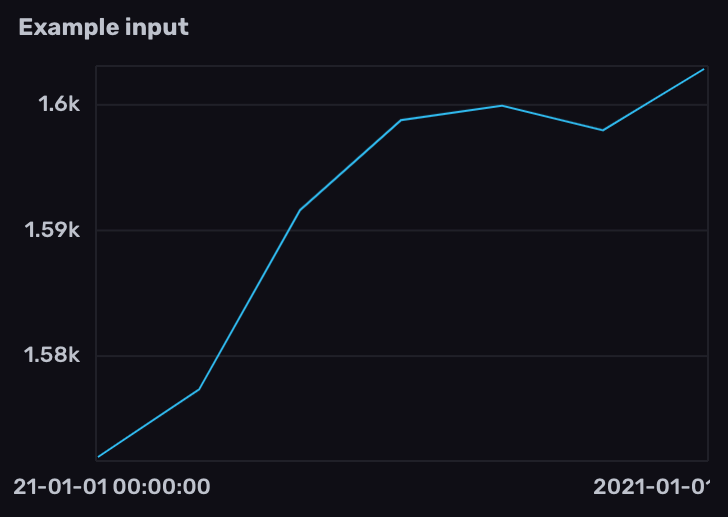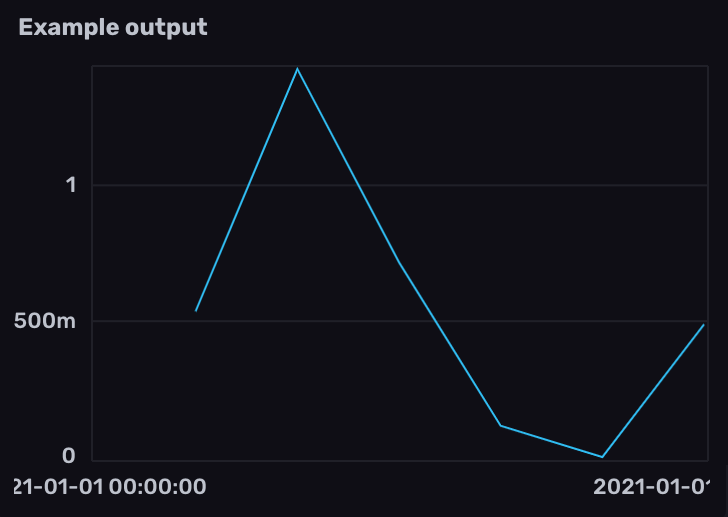# Work with Prometheus gauges

Use Flux to query and transform Prometheus gauge metrics stored in InfluxDB.

A gauge is a metric that represents a single numerical value that can arbitrarily go up and down.

Prometheus metric types

##### Example gauge metric in Prometheus data
``````# HELP example_gauge_current Current number of items as example gauge metric
# TYPE example_gauge_current gauge
example_gauge_current 128
``````

Generally gauge metrics can be used as they are reported and don’t require any additional processing.

The examples below include example data collected from the InfluxDB OSS 2.x `/metrics` endpoint using `prometheus.scrape()` and stored in InfluxDB.

#### Prometheus metric parsing formats

Query structure depends on the Prometheus metric parsing format used to scrape the Prometheus metrics. Select the appropriate metric format version below.

## Calculate the rate of change in gauge values

1. Filter results by the `prometheus` measurement and counter metric name field.
2. Use `derivative()` to calculate the rate of change between gauge values. By default, `derivative()` returns the rate of change per second. Use the `unit` parameter to customize the rate unit. To replace negative derivatives with null values, set the `nonNegative` parameter to `true`.
``````from(bucket: "example-bucket")
|> range(start: -1m)
|> filter(fn: (r) => r._measurement == "prometheus" and r._field == "go_goroutines")
|> derivative(nonNegative: true)
``````View example input and output data

1. Filter results by the counter metric name measurement and `gauge` field.
2. Use `derivative()` to calculate the rate of change between gauge values. By default, `derivative()` returns the rate of change per second. Use the `unit` parameter to customize the rate unit. To replace negative derivatives with null values, set the `nonNegative` parameter to `true`.
``````from(bucket: "example-bucket")
|> range(start: -1m)
|> filter(fn: (r) => r._measurement == "go_goroutines" and r._field == "gauge")
|> derivative(nonNegative: true)
``````View example input and output data

## Calculate the average rate of change in specified time windows

1. Import the `experimental/aggregate` package.

2. Filter results by the `prometheus` measurement and counter metric name field.

3. Use `aggregate.rate()` to calculate the average rate of change per time window.

``````import "experimental/aggregate"

from(bucket: "example-bucket")
|> range(start: -1m)
|> filter(fn: (r) => r._measurement == "prometheus" and r._field == "go_goroutines")
|> aggregate.rate(every: 10s, unit: 1s)
``````View example input and output data

1. Import the `experimental/aggregate` package.

2. Filter results by the counter metric name measurement and `gauge` field.

3. Use `aggregate.rate()` to calculate the average rate of change per time window.

``````import "experimental/aggregate"

from(bucket: "example-bucket")
|> range(start: -1m)
|> filter(fn: (r) => r._measurement == "go_goroutines" and r._field == "gauge")
|> aggregate.rate(every: 10s, unit: 1s)
``````View example input and output data

### Upgrade to InfluxDB Cloud or InfluxDB 2.0!

InfluxDB Cloud and InfluxDB OSS 2.0 ready for production.